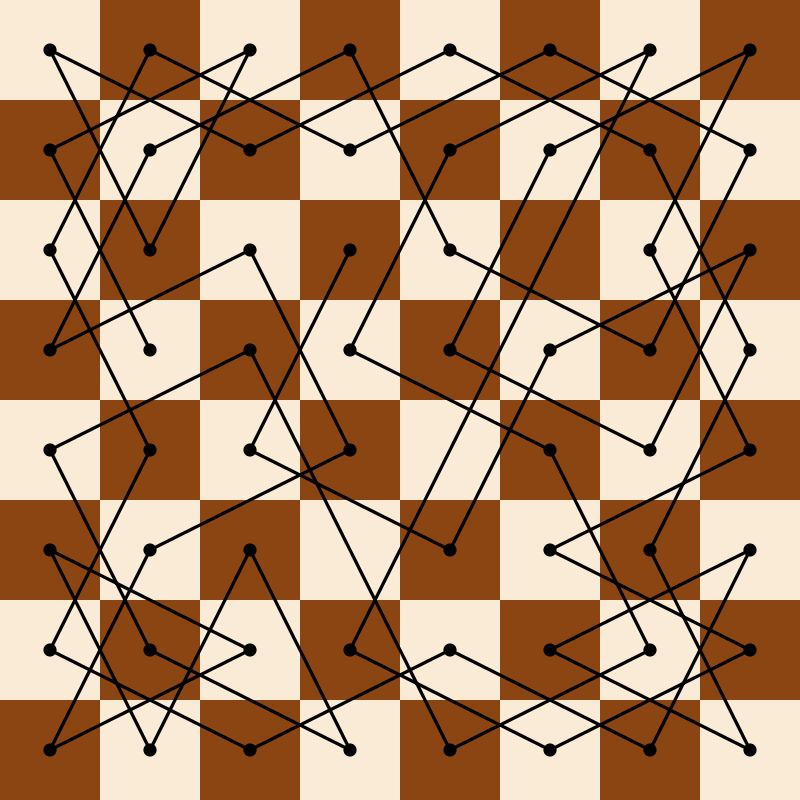Illustration of a knight tour on an 8x8 chessboard.Author: Brent Yorgey
> import Diagrams.Backend.SVG.CmdLine

A relatively well-known puzzle is to find a sequence of moves by which a knight can visit every square of a chessboard exactly once, without repeating any squares. This example computes such a tour and visualizes the solution.

> {-# LANGUAGE NoMonomorphismRestriction #-}
>
> import           Data.List                      (minimumBy, tails, (\\))
> import           Data.Ord                       (comparing)
> import           Diagrams.Prelude

First, we compute a tour by a brute force depth-first search (it does not take very long). This code is adapted from the code found here.

> type Square = (Int, Int)
>
> board :: [Square]
> board = [ (x,y) | x <- [0..7], y <- [0..7] ]
>
> knightMoves :: Square -> [Square]
> knightMoves (x,y) = filter (elem board) jumps
>   where jumps = [ (x+i,y+j) | i <- jv, j <- jv, abs i /= abs j ]
>         jv    = [1,-1,2,-2]
>
> knightTour :: Square -> [Square]
> knightTour sq = knightTour' [sq]
>   where
>     knightTour' moves@(lastMove:_)
>         | null candMoves = reverse moves
>         | otherwise = knightTour' $newSquare : moves > where newSquare = minimumBy (comparing (length . findMoves)) candMoves > candMoves = findMoves lastMove > findMoves s = knightMoves s \\ moves Now we can go about visualizing a tour. First, let’s draw a chessboard: > boardSq :: Colour Double -> Diagram B > boardSq c = square 1 # lw none # fc c > > chessBoard :: Int -> Diagram B > chessBoard n > = vcat . map hcat . map (map boardSq) > . take n . map (take n) . tails >$ cycle [antiquewhite, saddlebrown]

Now, we need a way to convert Square coordinates (a pair of numbers in the range 0-7) into actual coordinates on the chessboard. Since the chessboard ends up with its local origin in the center of the top-left square, all we need to do is negate the $$y$$-coordinate:

> squareToPoint :: Square -> P2 Double
> squareToPoint (x,y) = fromIntegral x ^& negate (fromIntegral y)

To draw a knight on a given square, we simply translate the given image appropriately:

> knight :: Square -> Diagram B -> Diagram B
> knight sq knightImg = knightImg # moveTo (squareToPoint sq)

Given a tour, we turn it into a path using fromVertices, and decorate the vertices with dots.

> drawTour :: [Square] -> Diagram B
> drawTour tour = tourPoints <> strokeP tourPath
>   where
>     tourPath   = fromVertices . map squareToPoint $tour > tourPoints = atPoints (concat . pathVertices$ tourPath) (repeat dot)
>     dot = circle 0.05 # fc black

Finally, we load a knight image, size it to fit a square, and then put all the previous pieces together:

> example = do
>   let knightImg = case res of
>         Left _    -> mempty
>         Right img -> image img # sized (mkWidth 1)
>   return \$ mconcat
>     [ knight tourStart knightImg
>     , knight tourEnd knightImg
>     , drawTour tour
>     , chessBoard 8
>     ]
>   where
>     tourStart = (1,3)
>     tour      = knightTour tourStart
>     tourEnd   = last tour
> main = mainWith (example :: Diagram B)